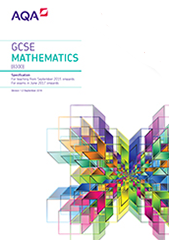# 3.1 Number

## 3.1.1 Structure and calculation

### N1

Basic foundation content

Higher content only

order positive and negative integers, decimals and fractions

use the symbols =, ≠, <, >, ≤, ≥

### N2

Basic foundation content

Higher content only

apply the four operations, including formal written methods, to integers, decimals and simple fractions (proper and improper), and mixed numbers – all both positive and negative

understand and use place value (eg when working with very large or very small numbers, and when calculating with decimals)

Notes: including questions set in context.

Knowledge and understanding of terms used in household finance, for example profit, loss, cost price, selling price, debit, credit, balance, income tax, VAT and interest rate. See also R9

### N3

Basic foundation content

Higher content only

recognise and use relationships between operations, including inverse operations (eg cancellation to simplify calculations and expressions)

use conventional notation for priority of operations, including brackets, powers, roots and reciprocals

### N4

Basic foundation content

Higher content only

use the concepts and vocabulary of prime numbers, factors (divisors), multiples, common factors, common multiples, highest common factor, lowest common multiple, prime factorisation, including using product notation and the unique factorisation theorem

Notes: prime factor decomposition including product of prime factors written in index form.

### N5

Basic foundation content

Higher content only

apply systematic listing strategies

including use of the product rule for counting

Notes: including using lists, tables and diagrams.

### N6

Basic foundation content

Higher content only

use positive integer powers and associated real roots (square, cube and higher), recognise powers of 2, 3, 4, 5

estimate powers and roots of any given positive number

Notes: including square numbers up to

Students should know that and 1 million =

### N7

Basic foundation content

Higher content only

calculate with roots, and with integer indices

calculate with fractional indices

### N8

Basic foundation content

Higher content only

calculate exactly with fractions

calculate exactly with multiples of

calculate exactly with surds

simplify surd expressions involving squares (eg ) and rationalise denominators

### N9

Basic foundation content

Higher content only

calculate with and interpret standard form, where and is an integer

Notes: with and without a calculator.

Interpret calculator displays.

## 3.1.2 Fractions, decimals and percentages

### N10

Basic foundation content

Higher content only

work interchangeably with terminating decimals and their corresponding fractions (such as 3.5 and or 0.375 and

change recurring decimals into their corresponding fractions and vice versa

Notes: including ordering.

### N11

Basic foundation content

Higher content only

identify and work with fractions in ratio problems

### N12

Basic foundation content

Higher content only

interpret fractions and percentages as operators

## 3.1.3 Measures and accuracy

### N13

Basic foundation content

Higher content only

use standard units of mass, length, time, money and other measures (including standard compound measures) using decimal quantities where appropriate

Notes: know and use metric conversion factors for length, area, volume and capacity.

Imperial/metric conversions will be given in the question.

### N14

Basic foundation content

Higher content only

check calculations using approximation and estimation, including answers obtained using technology

### N15

Basic foundation content

Higher content only

round numbers and measures to an appropriate degree of accuracy (eg to a specified number of decimal places or significant figures)

use inequality notation to specify simple error intervals due to truncation or rounding

Notes: including appropriate rounding for questions set in context.

Students should know not to round values during intermediate steps of a calculation. See also N14

### N16

Basic foundation content